Function Repository Resource:

# DirectionalDerivative

Compute the directional derivative of a function at a point

Contributed by: Dennis M Schneider
 ResourceFunction["DirectionalDerivative"][f,vars,pt,v] computes the directional derivative of the function f of the variables vars at the point pt in the direction of the normalized vector v.

## Details and Options

When the function is differentiable at a point (a1,a2,), then the directional derivative at (a1,a2,) in a direction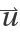can be calculated using the formula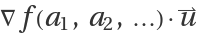where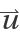is a unit vector.
The formulafor the directional derivative at a point (a1,a2,) requires that the function be differentiable at the point (a1,a2,). Setting the option "UseLimit" to true allows the computation of the directional derivative for non-differentiable functions.
If the formula is applied to a function whose gradient at a point is 0, then it will return 0 independent of the specified direction.

## Examples

### Basic Examples (2)

The directional derivative of a function of two variables at the point (1,-2) in the direction of the unit vector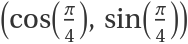:

 In:=Out=The directional derivative of the same function at a general point (a,b) in a general direction (cos(α),sin(α)):

 In:=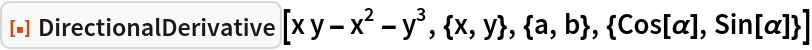Out=### Scope (6)

Find the directional derivative of a function of four variables:

 In:=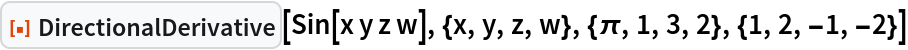Out=Since the following function is not differentiable at (0,0), it is necessary to use the limit definition to correctly calculate the directional derivative:

 In:=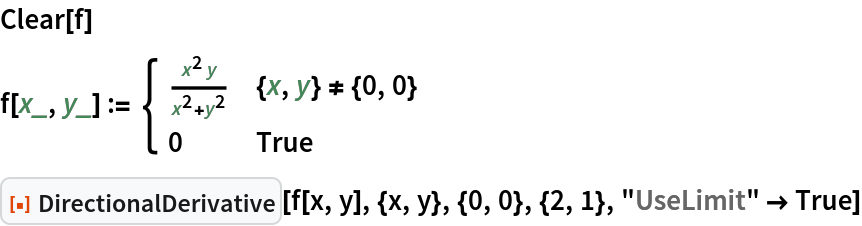Out=Calculate the gradient of this function at the origin:

 In:=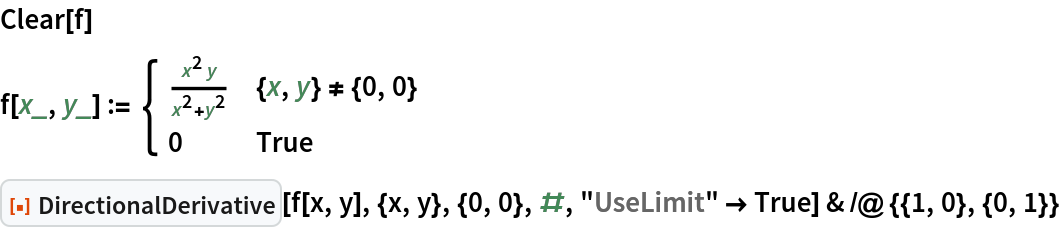Out=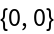In:=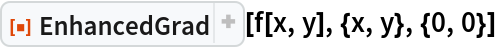Out=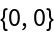Since the gradient of this function at (0,0) is (0,0), without setting "UseLimit"->True, the formula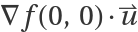returns 0 no matter the direction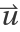:

 In:=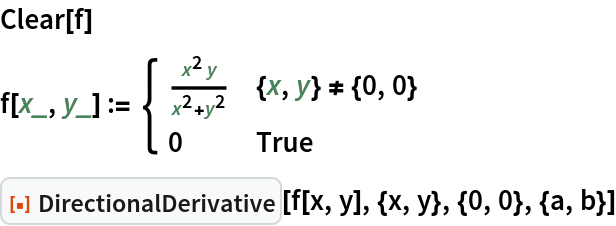Out=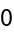A function that does not have a directional derivative except in the x and y directions:

 In:=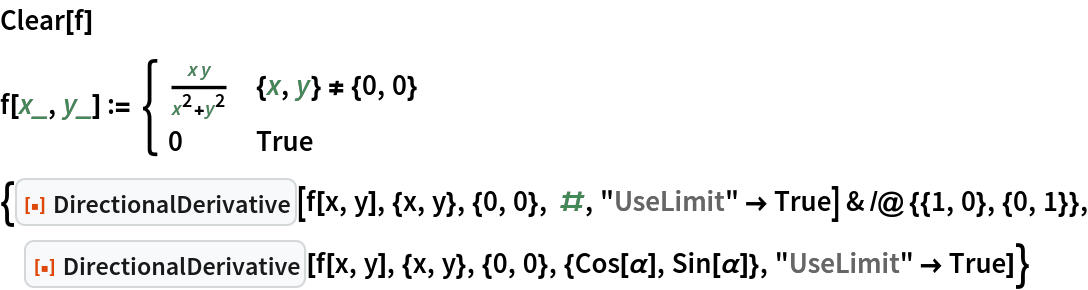Out=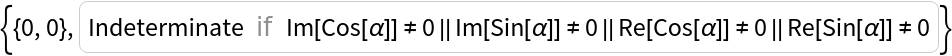A function requiring both "UseLimit" and "UseRealRoots":

 In:=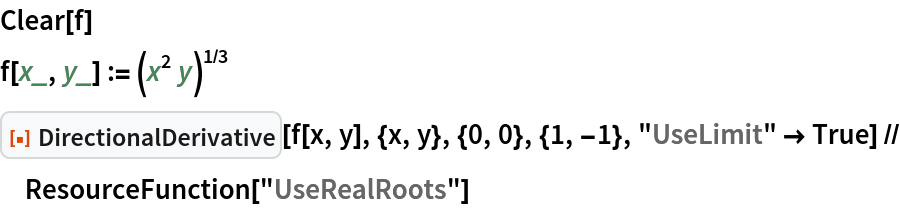Out=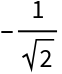Without using "UseRealRoots":

 In:=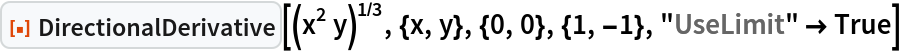Out=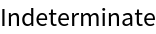Without using Limit:

 In:=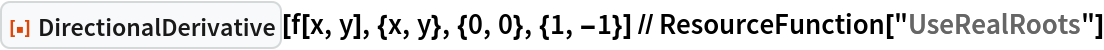Out=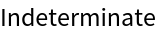## Publisher

Dennis M Schneider

## Version History

• 1.0.0 – 20 July 2022